# Shimura correspondence

By a modular form of weightone understands a functionon the upper half-plane satisfying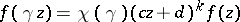for some suitable function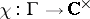when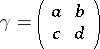is an element of some congruence subgroup of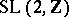(cf. also Modular function).

Ifis an integer, E. Hecke defined operators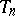for every integer, and showed they could be simultaneously diagonalizable (cf. also Hecke operator). The-series of a simultaneous eigenfunction (cf. also Dirichlet-function) is then an Euler product.

Modular forms of half-integral weight arise naturally, for example as theta-series. A theta-series invariables is a modular form of weight.

Ifis a half-integer,can only be defined ifis a square on forms of weight, and there is not enough information in the Hecke eigenvalues to determine the Fourier coefficients. The coefficients are not multiplicative, so the-series is not an Euler product.

Using the Rankin–Selberg method and a converse theorem, G. Shimura [a1] showed that ifis a modular form of weight, then there is a corresponding modular form of weightsuch that theHecke eigenvalue on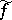agrees with theHecke eigenvalue of.

This result was complemented by the important theorem of J.-L. Waldspurger [a2], showing that theth Fourier coefficient ofagrees with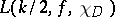. Waldspurger also gave interpretations of these special values as periods of(integrals over over geodesics). W. Kohnen and D. Zagier [a3] gave a particularly useful treatment of a special case. Also useful is [a4]. P. Sarnak and S. Katok [a5] found similar results for Maass forms.

Given Waldspurger's theorem, the case where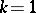becomes particularly interesting, since ifis the modular form of weight two associated with an elliptic curve,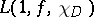has an interpretation in terms of the Birch–Swinnerton-Dyer conjecture. The period interpretation of the special values is then connected with the work of B.H. Gross, Kohnen and Zagier [a6] on heights of Heegner points. A beautiful application of this connection with the Birch–Swinnerton-Dyer conjecture to the classical problem of computing the set of areas of rational right triangles was given in [a7].

An interesting approach to the Shimura correspondence and Waldspurger's theorem is offered by the theory of Jacobi forms, in which bothand its correspondentmay be related to automorphic forms on the Jacobi group. See [a8] and [a9]; cf. also Automorphic form.

A. Weil realized that (Siegel) modular forms, particularly theta-series, should be interpreted as automorphic forms not on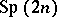, but on a certain double cover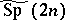, the so-called metaplectic group. If, then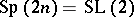, and this is the proper framework for understanding the classical Shimura correspondence, which can be regarded as a lifting from eitherto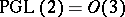, or from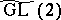to.

T. Kubota and K. Matsumoto constructed metaplectic covers of more general groups, provided the ground field contains sufficiently many roots of unity. The Shimura correspondence in this context is a lifting from automorphic forms on the covering group to automorphic forms onor (sometimes) its dual, obtained by reversing the long and short roots and interchanging the fundamental group with the dual of the centre. See [a10], [a11], [a12], [a13], [a14] for the Shimura correspondence on higher covers of higher rank groups. Finding analogues of Waldspurger's theorem in this context is an important open problem (as of 2000).

How to Cite This Entry:
Shimura correspondence. Encyclopedia of Mathematics. URL: http://encyclopediaofmath.org/index.php?title=Shimura_correspondence&oldid=36170
This article was adapted from an original article by D. Bump (originator), which appeared in Encyclopedia of Mathematics - ISBN 1402006098. See original article